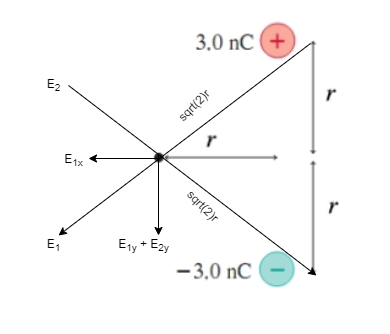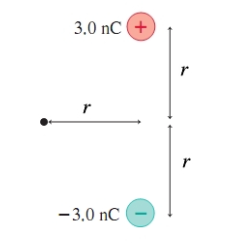# Problem: Part ASpecify the strength of the electric field at the point indicated by the dot. Let r = 6.5 cm.Part BSpecify the direction.

###### FREE Expert Solution

We can visualize the problem in the diagram below:d = sqrt(2)r = 0.09192 m

Part A

Electric field:

E = kq/d2###### Problem Details

Part A

Specify the strength of the electric field at the point indicated by the dot. Let r = 6.5 cm.

Part B

Specify the direction.